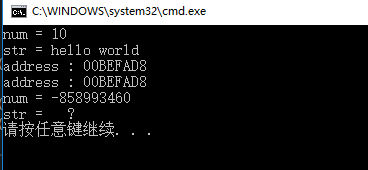C语言一个结构体指针的问题

`````` #include <stdio.h>

struct Test
{
int num;
char str;
};

struct Test * function();

int main()
{
struct Test *p = function();

printf("num = %d\n", p->num);
printf("str = %s\n", p->str);
}

struct Test * function()
{

struct Test test =
{
10,
"hello world"
};

struct Test *p = &test;

printf("num = %d\n", p->num);
printf("str = %s\n", p->str);

return p;
}

``````4个回答``````你返回的指针指向的内存已经释放了，用malloc或者是返回一个实体吧。
``````c语言 为什么函数可以返回了一个局部指针结构体？

C语言结构体指针的问题？

c语言基础的结构体嵌套问题

C语言截结构体指针中的变量又是另一个结构体指针，怎么用，代码运行不了

#include<stdio.h> #include<stdlib.h> #include<string.h> #include<malloc.h> #define LIST_INIT_SIZE 100//线性表存储空间的初始分配量 #define LISTINCREMENT 10//线性表存储空间的分配增量 typedef struct { int No; char name; }Student; typedef struct { Student *elem;//存储空间基址 int length;//当前啊长度 int listsize;//当前分配的存储容量 }SqList; int Initlist_Sq(SqList *L) { //构建一个空的线性表 printf("boy\n"); L->elem = (Student *)malloc(LIST_INIT_SIZE*sizeof(Student)); if(!L->elem) { printf("here\n"); return -2; } L->length = 0; L->listsize = LIST_INIT_SIZE; return 0; } int main() { SqList *L; int k = Initlist_Sq(L); int i; int n; printf("there\n"); if(k == -2) return -2; scanf("%d",&n); for(i = 0; i<n; i++) { L->elem[i].No=i; gets(L->elem[i].name); } return 0; }

C返回值是结构体指针问题

C语言 结构体指针字符 二进制文件写入和读取 指针字符输出错误

typedef struct s{ char *name; }student; /*将结构体读出并显示*/ int IsUsed(char *str) { FILE *fp = fopen("test","rb"); student s; s.name = (char*) malloc(sizeof(char)*20); fread(&s,sizeof(student),1,fp); while(!feof(fp)){ printf("%s",s.name); //为什么这里出错？？？？？？？？？？？？？？？？？？ if(strcmp(s.name,str)==0){ fclose(fp); return 1; //存在该字符串，则返回1 } fread(&s,sizeof(student),1,fp); } fclose(fp); return 0; //不存在该字符串，则返回0 } int main() { FILE *fp = fopen("test","rb+"); /*先将结构体P存入二进制文件中*/ student p; p.name = (char*) malloc(sizeof(char)*20); scanf("%s",p.name); while(IsUsed(p.name)){ //检查员工号是否唯一 printf("该字符串已经存在，请更换一个："); scanf("%s",p.name); } fwrite(&p,sizeof(student),1,fp); } 在printf哪里显示程序停止工作！！！！！！！！！求解啊

c语言 结构体指针输出信息不完整

#include<stdio.h> struct stu{ char *name; int num; int age; char group; float score; }stus = {{"Zhou ping", 5, 18, 'C', 145.0}, {"Zhang ping", 4, 19, 'A', 130.5}, {"Liu fang", 1, 18, 'A', 148.5}, {"Wang ming", 3, 17, 'B', 144.5} }, *p=stus; int main () { int j; int pjf ( struct stu w[],int i ); int charu ( struct stu a[],int n); pjf (stus,5); stus=stus; charu(stus,3); for(j=0;j<5;j++) { printf("%s,%d,%d,%c,%f\n",p[j].name,p[j].num,p[j].age,p[j].group,p[j].score); } return 0; } int pjf ( struct stu w[],int i ) { int sum=0; for(i=0;i<5;i++) { sum+=w[i].score; } printf ("%f\n",sum/4.0); return 0; } int charu ( struct stu a[],int n) { printf("请输入一条记录\n"); scanf("%s,%d,%d,%c,%f",&a.name,&a.num,&a.age,&a.group,&a.score); return 0; } ![图片说明](https://img-ask.csdn.net/upload/201703/08/1488959301_623774.png) 应该输出5组信息但是只有3组，不知道什么原因，跪求大神

c语言中结构体能否包含多个自身类型指针

C语言 socket 如何发送带指针的结构体？

String s = new String(" a ") 到底产生几个对象？

Linux面试题（2020最新版）

JVM内存结构和Java内存模型别再傻傻分不清了

loonggg读完需要3分钟速读仅需 1 分钟大家好，我是你们的校长。我之前讲过，这年头，只要肯动脑，肯行动，程序员凭借自己的技术，赚钱的方式还是有很多种的。仅仅靠在公司出卖自己的劳动时...

85后蒋凡：28岁实现财务自由、34岁成为阿里万亿电商帝国双掌门，他的人生底层逻辑是什么？...

MySQL数据库面试题（2020最新版）

HashMap底层实现原理，红黑树，B+树，B树的结构原理 Spring的AOP和IOC是什么？它们常见的使用场景有哪些？Spring事务，事务的属性，传播行为，数据库隔离级别 Spring和SpringMVC，MyBatis以及SpringBoot的注解分别有哪些？SpringMVC的工作原理，SpringBoot框架的优点，MyBatis框架的优点 SpringCould组件有哪些，他们...